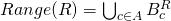# Basics of Relations

What's a relation?

Definition.  A relation from the set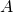to the set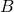is a(ny) subset of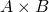. We callthe source andthe target or codomain of the relation.

A relation fromtois called a {\em relation on}.

If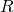is a relation fromto, and, we say that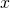is-related to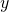, and sometimes write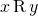. If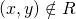, we sometimes write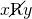.

The notationis called infix notation; the notation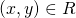is called set notation or postfix notation or reverse Polish notation. The term infix comes from linguistics. An infix is a grammatical feature that gets inserted into the middle of a word, as opposed to the beginning or the end. The examples of this in English are almost all obscenities. The term reverse Polish refers to the school of logicians founded by Jan Łukasiewicz and Kazimierz Twardowski at the Universities of Lwów and Warsaw in the early 1900s.

Exercise. A relation on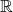is a subset of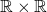. What is a relation on?

Eg. The identity relation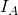on any setis defined by: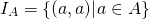Thenmeans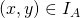, i.e. that there is some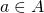with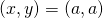. But then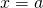and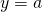, so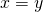.

Thus the identity relation is nothing more than equality.

If we draw a picture of the identity relation, we get a diagonal line, so the identity relation is sometimes also called the  diagonal relation on.

Exercise. Ifhas exactlyelements andhas exactly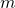elements, how many relations are there fromto?

Since relations are sets, we can do set operations to them (although this is usually not so interesting), for example:

Eg. Consider the relation ongiven by: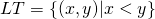Then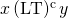iff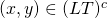iff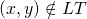iff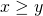.

We could summarize this all by saying  the complement of the less-than relation is the greater-than-or-equal-to relation.

Note thathas a fairly natural meaning in terms of relationships: to say two elements are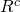-related is precisely to say they are not-related.

Exercise. Explain what the intersection and union of two relations would mean.

### Domain, Range, and Fibers of a Relation

To any relationfrom a setto a set, we can associate the following sets:

Definition. The domain ofis the set of its first coordinates. That is:The range ofis the set of its second coordinates. That is: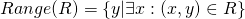Observe that the domain is automatically a subset of the source, and the range is automatically a subset of the target. We can also focus on particular first coordinates:

Definition. The horizontal fiber at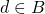is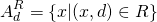The vertical fiber at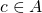is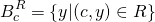That is, the horizontal fiber is all the first coordinates with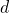as their corresponding second coordinate.

Theorem. The domain and the range are unions of the corresponding fibers. That is,

•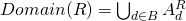•